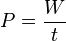# Power (physics) facts for kids

Kids Encyclopedia Facts

In physics, power (symbol: P) is how fast work is done or energy is given from one thing to another. In the SI system of measurement, power is measured in watts (symbol: W).

It may take a certain amount of work to make a change in the world, such as lifting a heavy weight to a higher level. It makes no difference if the weight is lifted slowly or quickly. The same amount of work will have been performed. The difference between a slow lift and a quick lift is that a quick lift requires more power. This means the work can be done in a shorter time if there is more power available.

As a rate of change of work done or the energy of a subsystem, power is:$P=\frac{W}{t}$

where

P is power
W is work
t is time.

Power is also equal to force times velocity (or speed).

## Power in optics

In optics, or radiometry, the term power sometimes refers to radiant flux. This is the average rate of energy transport by electromagnetic radiation, measured in watts. But the term "power" is also used to express the ability of a lens or other optical device to focus light. It is measured in dioptres (inverse metres), and is equal to one over the focal length of the optical device.

## Images for kidsPower (physics) Facts for Kids. Kiddle Encyclopedia.